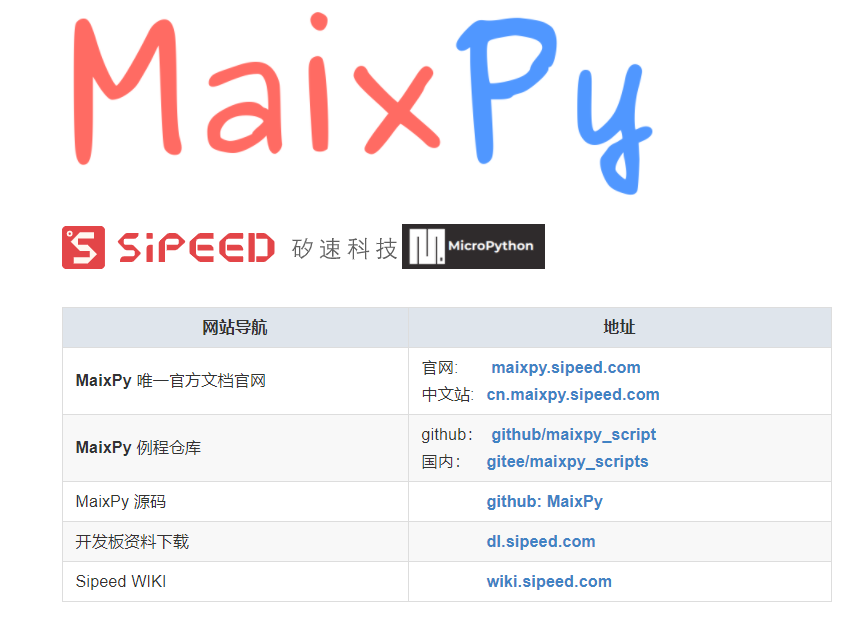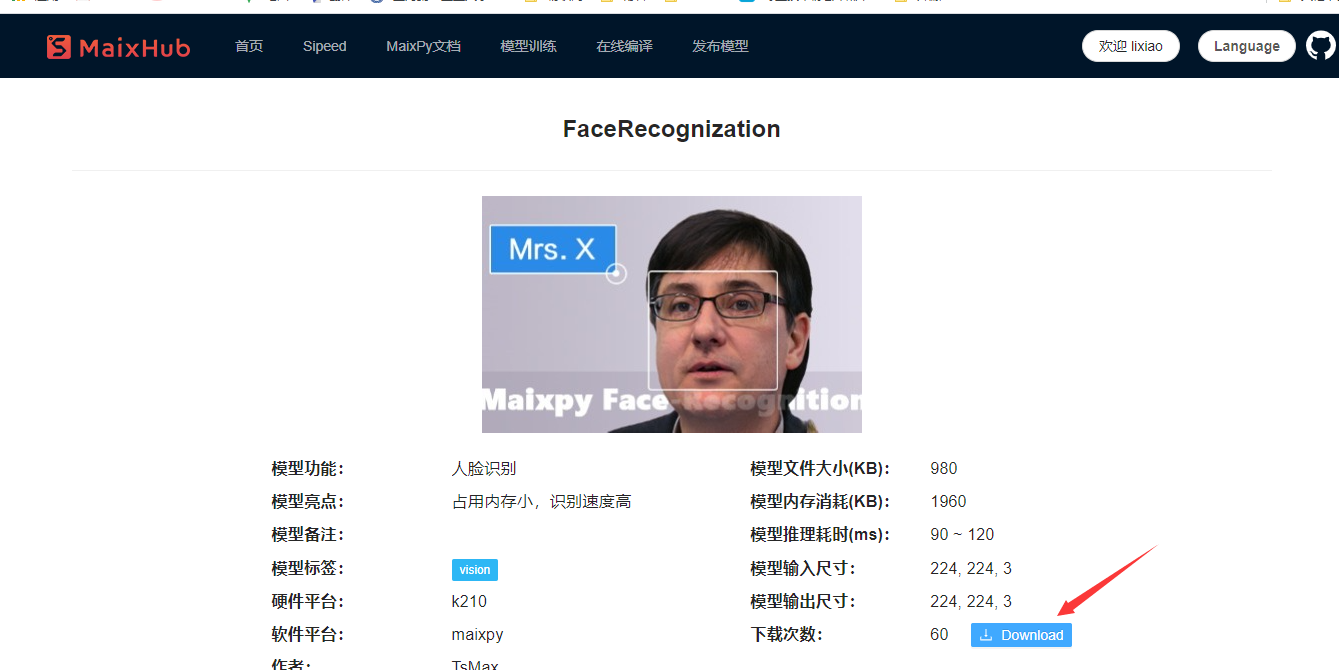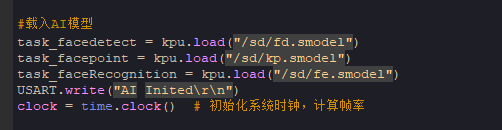# K210开发板学习笔记（三）——STM32+K210+SD卡实现人脸识别（完整K210代码）1、配置步骤如下**注意：**使用亚博智能K210开发板时，Maixpy IDE中工具选择开发板，选Kendryte KD233开发板就可以使用Maixpy IDE开发亚博的板子了。
2.、程序理解STM32和K210之间我采取串口通信的方式，STM32那边我配置的是串口2，波特率设置成115200，K210部分设置TX和RX引脚，在调用串口初始化函数即可，使用杜邦线连接对应的引脚，这里注意串口通信要反接，TX脚连接的是RX脚，RX脚连接的是TX脚。

STM32代码部分如下

``````//这里我主要是采用if判断的方式发送命令给K210
void bsp_k210 (void){
if(getvalueuart==0x12){
Usart_SendByte(USART2,'A');//存储式录入人脸
}else if(getvalueuart==0x13)
{
Usart_SendByte(USART2,'C');//临时存储录入人脸
}
else// if(getvalueuart==14)
{
;//Usart_SendByte(USART2,'S');//切换模型，硬件重启，可以实现口罩检测部分
}
}
``````

K210代码部分如下

``````import sensor,image,lcd  # import 相关库
import KPU as kpu
import time
import os
from Maix import FPIOA,GPIO
from fpioa_manager import fm
import ubinascii
from machine import UART
from machine import Timer
import time

fm.register(23,fm.fpioa.UART1_TX)#串口引脚映射
fm.register(19,fm.fpioa.UART1_RX)#这两个引脚是可以任意修改的

com = UART(UART.UART1, 115200, timeout=50, read_buf_len=4096)#构建串口对象

#定时器中断回调函数
tem = ''
b = []
check = 0  #存储
save = 0   #临时存储
switch = 0 #切换
def on_timer(timer):  #回调函数
global check
global save
data = []
if data!=None:
print(data)
check = 1#代表存储人脸特征
if data == b'A':
save = 0  #不存到SD卡中
elif data == b'C':
save = 1  #存到SD卡中
print(data)
#定时器中断初始化
tim = Timer(Timer.TIMER0, Timer.CHANNEL0, mode=Timer.MODE_ONE_SHOT, period=500,
unit=Timer.UNIT_MS,callback=on_timer, arg=on_timer,start=False)

feature_file_exists = 0
for v in os.ilistdir('/sd'):#to check key directorys or files in sd card.sd card should be formated to fat32
if v == 'features.txt' and v == 0x8000:#0x8000 is file
feature_file_exists = 1

record_ftr=[] #空列表 用于存储当前196维特征
record_ftrs=[] #空列表 用于存储按键记录下人脸特征， 可以将特征以txt等文件形式保存到sd卡后，读取到此列表，即可实现人脸断电存储。
names = ['LX', 'HLF', 'MR.3', 'Mr.4', 'Mr.5', 'Mr.6', 'Mr.7', 'Mr.8', 'Mr.9' , 'Mr.10'] # 人名标签，与上面列表特征值一一对应。
reco = ''
record = []
def save_feature(feat):
with open('/sd/features.txt','a') as f:
record =ubinascii.b2a_base64(feat)
f.write(record)

st = ''
if(feature_file_exists):
print("start")
with open('/sd/features.txt','rb') as f:
print(len(s))
#print(s)
for line in s:
#print(ubinascii.a2b_base64(line))
record_ftrs.append(bytearray(ubinascii.a2b_base64(line)))

print(record_ftr,names)

print(len(record_ftr))
print("end")

task_fd = kpu.load(0x300000) # 从flash 0x200000 加载人脸检测模型
task_ld = kpu.load(0x400000) # 从flash 0x300000 加载人脸五点关键点检测模型
task_fe = kpu.load(0x500000) # 从flash 0x400000 加载人脸196维特征值模型
clock = time.clock()  # 初始化系统时钟，计算帧率

lcd.init() # 初始化lcd
sensor.reset() #初始化sensor 摄像头
sensor.set_pixformat(sensor.RGB565)
sensor.set_framesize(sensor.QVGA)
sensor.set_hmirror(1) #设置摄像头镜像
sensor.set_vflip(1)   #设置摄像头翻转
lcd.rotation()
sensor.run(1) #使能摄像头

anchor = (1.889, 2.5245, 2.9465, 3.94056, 3.99987, 5.3658, 5.155437, 6.92275, 6.718375, 9.01025) #anchor for face detect 用于人脸检测的Anchor
dst_point = [(44,59),(84,59),(64,82),(47,105),(81,105)] #standard face key point position 标准正脸的5关键点坐标 分别为 左眼 右眼 鼻子 左嘴角 右嘴角
a = kpu.init_yolo2(task_fd, 0.5, 0.3, 5, anchor) #初始化人脸检测模型
img_lcd=image.Image() # 设置显示buf
img_face=image.Image(size=(128,128)) #设置 128 * 128 人脸图片buf
a=img_face.pix_to_ai() # 将图片转为kpu接受的格式

while(1): # 主循环
check = 0
save = 0
tim.start()  #定时器中断开始
img = sensor.snapshot() #从摄像头获取一张图片
clock.tick() #记录时刻，用于计算帧率
code = kpu.run_yolo2(task_fd, img) # 运行人脸检测模型，获取人脸坐标位置
#b = img.draw_string(0,0, ("tem"), color=(0,255,0),scale=2)
if code: # 如果检测到人脸
for i in code: # 迭代坐标框
# Cut face and resize to 128x128
a = img.draw_rectangle(i.rect()) # 在屏幕显示人脸方框
face_cut=img.cut(i.x(),i.y(),i.w(),i.h()) # 裁剪人脸部分图片到 face_cut
face_cut_128=face_cut.resize(128,128) # 将裁出的人脸图片 缩放到128 * 128像素
a=face_cut_128.pix_to_ai() # 将猜出图片转换为kpu接受的格式
#a = img.draw_image(face_cut_128, (0,0))
# Landmark for face 5 points
fmap = kpu.forward(task_ld, face_cut_128) # 运行人脸5点关键点检测模型
plist=fmap[:] # 获取关键点预测结果
le=(i.x()+int(plist*i.w() - 10), i.y()+int(plist*i.h())) # 计算左眼位置， 这里在w方向-10 用来补偿模型转换带来的精度损失
re=(i.x()+int(plist*i.w()), i.y()+int(plist*i.h())) # 计算右眼位置
nose=(i.x()+int(plist*i.w()), i.y()+int(plist*i.h())) #计算鼻子位置
lm=(i.x()+int(plist*i.w()), i.y()+int(plist*i.h())) #计算左嘴角位置
rm=(i.x()+int(plist*i.w()), i.y()+int(plist*i.h())) #右嘴角位置
a = img.draw_circle(le, le, 4)
a = img.draw_circle(re, re, 4)
a = img.draw_circle(nose, nose, 4)
a = img.draw_circle(lm, lm, 4)
a = img.draw_circle(rm, rm, 4) # 在相应位置处画小圆圈
# align face to standard position
src_point = [le, re, nose, lm, rm] # 图片中 5 坐标的位置
T=image.get_affine_transform(src_point, dst_point) # 根据获得的5点坐标与标准正脸坐标获取仿射变换矩阵
a=image.warp_affine_ai(img, img_face, T) #对原始图片人脸图片进行仿射变换，变换为正脸图像
a=img_face.ai_to_pix() # 将正脸图像转为kpu格式
#a = img.draw_image(img_face, (128,0))
del(face_cut_128) # 释放裁剪人脸部分图片
# calculate face feature vector
fmap = kpu.forward(task_fe, img_face) # 计算正脸图片的196维特征值
feature=kpu.face_encode(fmap[:]) #获取计算结果
reg_flag = False
scores = [] # 存储特征比对分数
for j in range(len(record_ftrs)): #迭代已存特征值
score = kpu.face_compare(record_ftrs[j], feature) #计算当前人脸特征值与已存特征值的分数
scores.append(score) #添加分数总表
max_score = 0
index = 0
for k in range(len(scores)): #迭代所有比对分数，找到最大分数和索引值
if max_score < scores[k]:
max_score = scores[k]
index = k
if max_score > 80: # 如果最大分数大于85， 可以被认定为同一个人
a = img.draw_string(i.x(),i.y(), ("%s :%2.1f" % (names[index], max_score)), color=(0,255,0),scale=2) # 显示人名 与 分数
else:
a = img.draw_string(i.x(),i.y(), ("X :%2.1f" % (max_score)), color=(255,0,0),scale=2) #显示未知 与 分数
if check ==1: #如果检测到串口数据
check = 0
record_ftr = feature
record_ftrs.append(record_ftr) #将当前特征添加到已知特征列表
if save== 1:  #如果存储
save = 0
a = img.draw_string(100,100, "Stor successful", color=(0,255,0),scale=2)
print("stor successful")
save_feature(record_ftr) #存到SD卡
break
a = img.draw_string(0,220,"face", color=(255,0,0),scale=2) #显示未知 与 分数
a = lcd.display(img) #刷屏显示

``````